## RS Aggarwal Class 8 Solutions Chapter 25 Graphs Ex 25C

These Solutions are part of RS Aggarwal Solutions Class 8. Here we have given RS Aggarwal Solutions Class 8 Chapter 25 Graphs Ex 25C.

Other Exercises

OBJECTIVE QUESTIONS
Tick the correct answer in each of the following:

Question 1.
Solution:
∵ In point (3, 6), both x and y are positive.

Question 2.
Solution:
∵ In point ( – 7, – 1) both x and y are negative.

Question 3.
Solution:
∵ In point (2, – 3), x is positive and y is negative.

Question 4.
Solution:
∵ In point ( – 4, 1), x is negative and y is positive.

Question 5.
Solution:
∵ Abscissa is distance of a point from y- axis

Question 6.
Solution:
y = a is a line parallel to x-axis at a distance of ‘a’ units.

Question 7.
Solution:
The equation of the line y-axis, is x = 0

Hope given RS Aggarwal Solutions Class 8 Chapter 25 Graphs Ex 25C are helpful to complete your math homework.

If you have any doubts, please comment below. Learn Insta try to provide online math tutoring for you.

## RS Aggarwal Class 8 Solutions Chapter 25 Graphs Ex 25B

These Solutions are part of RS Aggarwal Solutions Class 8. Here we have given RS Aggarwal Solutions Class 8 Chapter 25 Graphs Ex 25B.

Other Exercises

Question 1.
Solution:
(a) y = 3x
By giving some different values to x, we shall get corresponding values of y.
x = 1 then y = 3 x 1 = 3
if x = 2, then y = 3 x 2 = 6
if x = 0, then y = 3 x 0 = 0Now plotting the points given above, and joining them.
(b) We get a line, From the graph.
(i) When x = 3, then y = 9
(ii) When x = 5, then y = 15
(iii) When x = 6, then y = 18 Ans.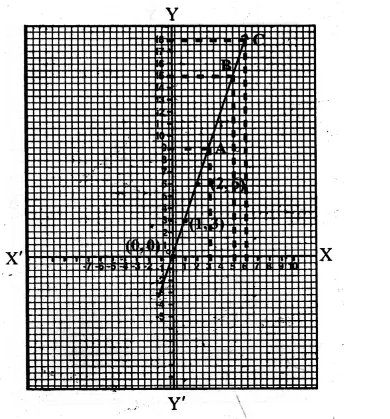Question 2.
Solution:
(a) P = 4x
By giving some different values to x, we get the corresponding values of y or P
If x = 1, then P = 4 x 1 = 4
if x = 2, then P = 4 x 2 = 8
if x = 0, then P = 4 x 0 = 0Plot the points (1, 4), (2, 8) and 0, 0) on the graph and join then to get the graph of P = 4x as shown
(b) From the graph we see that
(i) When x = 3, then P = 12
(ii) When x = 4, then P = 16
(iii) When x = 6, then P = 24 Ans.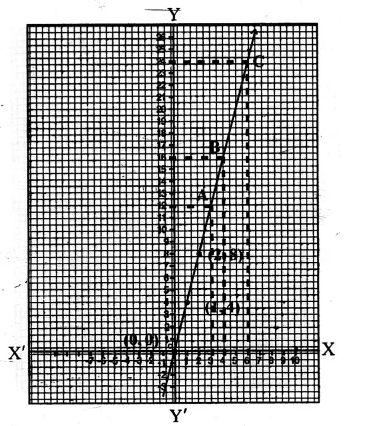Question 3.
Solution:
A = x²
giving some values to x, we get corresponding values of y or A
If x = 1, then y or A = (1)² = 1
If x = 2, then y or A = (2)² = 4
If x = 0, then y or A = (0)² = 0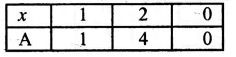Now plot the point (1, 1), (2, 4), (0, 0) on the graph, and join them to get the graph of A = x2 as shown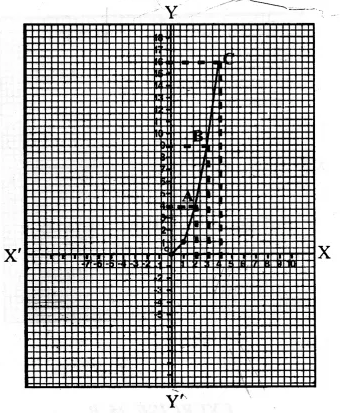(b) From the graph we see that
(i) When x = 2, then A = 4
(ii) When x = 3, then A = 9
(ii) When x = 4 then A = 16 Ans.

Hope given RS Aggarwal Solutions Class 8 Chapter 25 Graphs Ex 25B are helpful to complete your math homework.

If you have any doubts, please comment below. Learn Insta try to provide online math tutoring for you.

## RS Aggarwal Class 8 Solutions Chapter 25 Graphs Ex 25A

These Solutions are part of RS Aggarwal Solutions Class 8. Here we have given RS Aggarwal Solutions Class 8 Chapter 25 Graphs Ex 25A.

Other Exercises

Question 1.
Solution:
Below is given the graph in which X’OX and YOY’ are the co-ordinate axes intersecting each other at O. Now the. points given have been plotted as shown on the graph.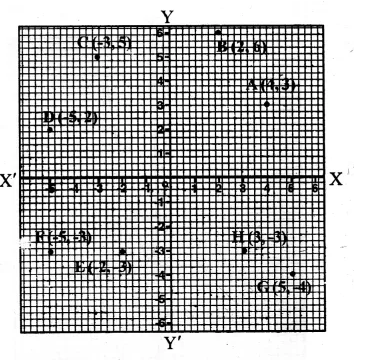Hope given RS Aggarwal Solutions Class 8 Chapter 25 Graphs Ex 25A are helpful to complete your math homework.

If you have any doubts, please comment below. Learn Insta try to provide online math tutoring for you.

## RS Aggarwal Class 8 Solutions Chapter 24 Probability Ex 24B

These Solutions are part of RS Aggarwal Solutions Class 8. Here we have given RS Aggarwal Solutions Class 8 Chapter 24 Probability Ex 24B.

Other Exercises

Tick the correct answer in each of the following :

Question 1.
Solution:
A spinning wheel has 3 white and 5 green sectors
Possible out come = 3 + 5 = 8
It is spinners, then
Probability of getting a green sector = $$\\ \frac { 5 }{ 8 }$$ (b)

Question 2.
Solution:
8 cards are numbered 1, 2, 3, 4, 5, 6, 7, 8
They are mixed and kept in a box One card is chosen at random, then Probability of card having 9 number less than 4 = $$\\ \frac { 3 }{ 8 }$$ (c)

Question 3.
Solution:
Two coins are tossed simultaneously, then Possible outcomes = 4
Now probability of getting one head and one tail = $$\\ \frac { 2 }{ 4 }$$ = $$\\ \frac { 1 }{ 2 }$$ (b)

Question 4.
Solution:
. In a bag, there are 3 white and 2 red balls
Possible outcomes = 3 + 2 = 5
Now probability of a red ball drawn
= $$\\ \frac { 2 }{ 5 }$$ (d)

Question 5.
Solution:
A die is thrown then
Possible outcomes = 6
Now probability of getting 6 is $$\\ \frac { 1 }{ 6 }$$ (b)

Question 6.
Solution:
A die is thrown
Possible outcomes = 6
Now probability of getting an even number
which are 2, 4, 6 = $$\\ \frac { 3 }{ 6 }$$ = $$\\ \frac { 1 }{ 2 }$$ (a)

Question 7.
Solution:
One card is drawn from a well shuffled deck of 52 cards, possible out comes = 52
The probability of card which is a queen = $$\\ \frac { 4 }{ 52 }$$
= $$\\ \frac { 1 }{ 13 }$$ (c)

Question 8.
Solution:
One card is drawn from a well-shuffled deck of 52 card, possible out comes = 52 Probability of a card being a black 6
(which are two) = $$\\ \frac { 2 }{ 52 }$$ = $$\\ \frac { 1 }{ 26 }$$ (b)

Hope given RS Aggarwal Solutions Class 8 Chapter 24 Probability Ex 24B are helpful to complete your math homework.

If you have any doubts, please comment below. Learn Insta try to provide online math tutoring for you.

## RS Aggarwal Class 8 Solutions Chapter 24 Probability Ex 24A

These Solutions are part of RS Aggarwal Solutions Class 8. Here we have given RS Aggarwal Solutions Class 8 Chapter 24 Probability Ex 24A.

Other Exercises

Question 1.
Solution:
(i) When a coin is tossed, we get outcomes 2 as H or T (Head or Tail)
(ii) When two coins are tossed together, we get possible four outcomes as HH, HT, TH, TT
(iii) A die is thrown, we get possible outcomes as 1,2, 3, 4, 5, 6
(iv) From a well – shuffled deck of 52 cards, 0ne card is at random drawn, we get the possible outcomes is 52

Question 2.
Solution:
Possible outcomes = 2
In a single throw of a coin, we get
probability of getting a tail = $$\\ \frac { 1 }{ 2 }$$

Question 3.
Solution:
In a single throw of two coins, possible outcomes = 4
(i) Probability of getting both tails = $$\\ \frac { 1 }{ 4 }$$
(ii) Probability of getting at least one tail = $$\\ \frac { 3 }{ 4 }$$
(iii) Probability of getting at the most one tail = $$\\ \frac { 2 }{ 4 }$$ = $$\\ \frac { 1 }{ 2 }$$

Question 4.
Solution:
In a bag, there are 4 white and 5 blue balls ,
Possible outcomes = 4 + 5 = 9
One ball is drawn at random, then
(i) the probability of a white ball = $$\\ \frac { 4 }{ 9 }$$
(ii) the probability of a blue ball = $$\\ \frac { 5 }{ 9 }$$

Question 5.
Solution:
In a bag, there are 5 white, 6 red and 4 green balls
Possible outcome is 5 + 6 + 4 = 15
One ball is drawn at random, then
(i) Probability of a green ball = $$\\ \frac { 4 }{ 15 }$$
(ii) Probability of a white ball = $$\\ \frac { 5 }{ 15 }$$ = $$\\ \frac { 1 }{ 3 }$$
(iii) Probability of a non-red ball = $$\\ \frac { 5+4 }{ 15 }$$
= $$\\ \frac { 9 }{ 15 }$$
= $$\\ \frac { 3 }{ 5 }$$
(5 white and 4 green balls are non-red balls)

Question 6.
Solution:
In a lottery, there are 10 prizes and 20 blanks
Possible outcomes = 10 + 20 = 30
A ticket is chosen at random, then
probability of getting a prize = $$\\ \frac { 10 }{ 30 }$$ = $$\\ \frac { 1 }{ 3 }$$

Question 7.
Solution:
In a ,box of 100 electric bulb, 8 are defective
Then non-defective bulbs = 100 – 8 = 92
Now possible outcomes = 100
(i) Probability of a drawn bulb, which is defective = $$\\ \frac { 8 }{ 100 }$$ = $$\\ \frac { 2 }{ 25 }$$
(ii) Probability of a drawn bulb which is non defective = $$\\ \frac { 92 }{ 100 }$$ = $$\\ \frac { 23 }{ 25 }$$

Question 8.
Solution:
A die is thrown, then
Possible outcomes = 6
(i) Now probability of getting 2 = $$\\ \frac { 1 }{ 6 }$$
(ii) Probability of a number less than 3 (which are 1 and 2) = $$\\ \frac { 2 }{ 6 }$$ = $$\\ \frac { 1 }{ 3 }$$
(iii) Probability of a composite number (a composite number is a number which is not a prime number which are 4, 6) = $$\\ \frac { 2 }{ 6 }$$ = $$\\ \frac { 1 }{ 3 }$$
(iv) Probability of a number not less than 4 (which are 5, 6) = $$\\ \frac { 2 }{ 6 }$$ = $$\\ \frac { 1 }{ 3 }$$

Question 9.
Solution:
Total number of ladies = 200
Those who like coffee = 82
Those who dislike coffee = 118
Possible number of outcomes = 200
One lady is chosen at random, then
(i) Probability of a lady who dislikes coffee = $$\\ \frac { 118 }{ 200 }$$
= $$\\ \frac { 59 }{ 100 }$$

Question 10.
Solution:
19 ball bearing numbers, 1, 2, 3,…19
possible outcomes = 19
A ball is drawn at random from the box, then
(i) Probability of a ball which bears a prime numbers which are 2, 3, 5, 7, 11, 13, 17 and 19 = 8 = $$\\ \frac { 8 }{ 19 }$$
(ii) Probability of a ball which bears an even number which are 2, 4, 6, 8, 10, 12, 14, 16, 18 = 9 = $$\\ \frac { 9 }{ 19 }$$
(iii) Probability of a number which bears a number divisible by 3 which are 3, 6, 9, 12, 15, 18 = 6 = $$\\ \frac { 6 }{ 19 }$$

Question 11.
Solution:
A card’s drawn at random from a deck
of well-shuffled deck of 52 cards Probability = 52
(i) Probability of a card being a king = $$\\ \frac { 4 }{ 52 }$$ = $$\\ \frac { 1 }{ 13 }$$
(ii) Probability of a card being spade = $$\\ \frac { 13 }{ 52 }$$ = $$\\ \frac { 1 }{ 4 }$$
(iii) Probability of a card being a red queen = $$\\ \frac { 2 }{ 52 }$$ = $$\\ \frac { 1 }{ 26 }$$
(iv) Probability of a card being a black 8 = $$\\ \frac { 2 }{ 52 }$$ = $$\\ \frac { 1 }{ 26 }$$

Question 12.
Solution:
One card is drawn at random from a deck of well shuffled deck of 52 cards
Possible outcomes = 52
(i) Probability of a card being a 4 = $$\\ \frac { 4 }{ 52 }$$ = $$\\ \frac { 1 }{ 13 }$$
(ii) Probability of a card being a queen = $$\\ \frac { 4 }{ 52 }$$ = $$\\ \frac { 1 }{ 13 }$$
(iii) Probability of a card being a black card = $$\\ \frac { 26 }{ 52 }$$ = $$\\ \frac { 1 }{ 2 }$$

Hope given RS Aggarwal Solutions Class 8 Chapter 24 Probability Ex 24A are helpful to complete your math homework.

If you have any doubts, please comment below. Learn Insta try to provide online math tutoring for you.

## RS Aggarwal Class 8 Solutions Chapter 23 Pie Charts Ex 23B

These Solutions are part of RS Aggarwal Solutions Class 8. Here we have given RS Aggarwal Solutions Class 8 Chapter 23 Pie Charts Ex 23B.

Other Exercises

OBJECTIVE QUESTIONS :
Tick the correct answer in each of the following:

Question 1.
Solution:
Central angles = $$\\ \frac { 250 }{ 2400 }$$ x 360°
= $$\\ \frac { 75 }{ 2 }$$
= $$37{ \frac { 1 }{ 2 } }^{ o }$$

Question 2.
Solution:
Central angle = $$\\ \frac { 35 }{ 100 }$$ x 360°
= 126°

Question 3.
Solution:
Total number of strength = 1650
Arts stream’s central angle = 48°
No. of students of Arts stream
= $$\\ \frac { 48 }{ 360 }$$ x 1650
= 220

Question 4.
Solution:
Central angle of students reading novels = 81°
$$\\ \frac { 81 }{ 360 }$$ x 100
= $$\\ \frac { 45 }{ 2 }$$
= $$22{ \frac { 1 }{ 2 } }$$%

Hope given RS Aggarwal Solutions Class 8 Chapter 23 Pie Charts Ex 23B are helpful to complete your math homework.

If you have any doubts, please comment below. Learn Insta try to provide online math tutoring for you.

## RS Aggarwal Class 8 Solutions Chapter 23 Pie Charts Ex 23A

These Solutions are part of RS Aggarwal Solutions Class 8. Here we have given RS Aggarwal Solutions Class 8 Chapter 23 Pie Charts Ex 23A.

Other Exercises

Question 1.
Solution:
Total expenditure = Rs. 4000 + 5400 + 2800 + 1800 + 400 = Rs. 14400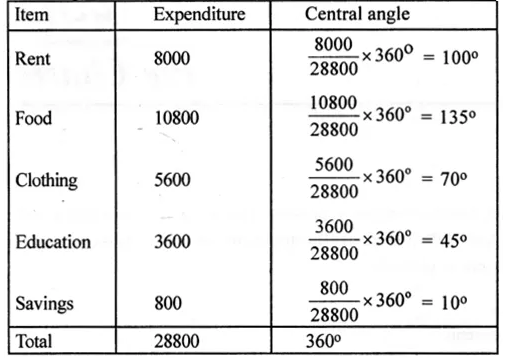Construction of pie chart :
1. Draw a circle of any convenient radius.
3. Staring from this radius, draw sectors of central angle 100°, 135°, 70°, 45° and 10° respectively.
4. Shade these sectors with different colors or designs as shown in the figure.
This is the required pie chart.Question 2.
Solution:
Total number of creatures 900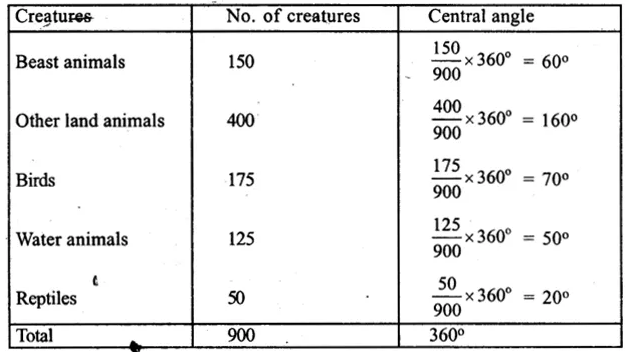(i) Draw a circle with a suitable radius.
(iii) Starting from this radius, draw sectors whose central angles are 60°, 160°, 70°, 50° and 20° respectively.
(iv) Now shade each sector with different colours or designs as shown in the figure.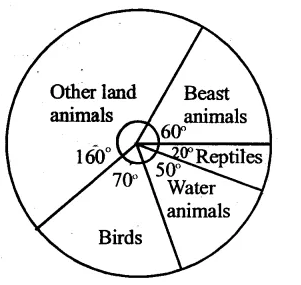Question 3.
Solution:
Total number of students = 350 + 245 + 210 + 175 + 280 = 1260(i) Draw a circle with a suitable radius.
(iii) Starting from this radius draw sectors whose central angles are 100°, 70°, 60°, 50° and 80° respectively.
(iv) Now shade each sector with different colours or designs as shown in the figure.Question 4.
Solution:(i) Draw a circle with a suitable radius.
(iii) Starting from this radius, draw sectors whose actual angles are 105°, 60°, 30°, 120° and 45° respectively.
(iv) Now shade each sector with different colours or design as shown in the figure.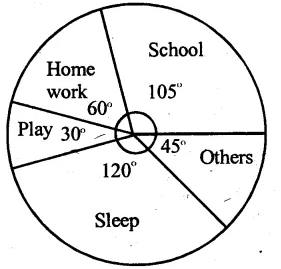Question 5.
Solution:
Here total number of workers = 1080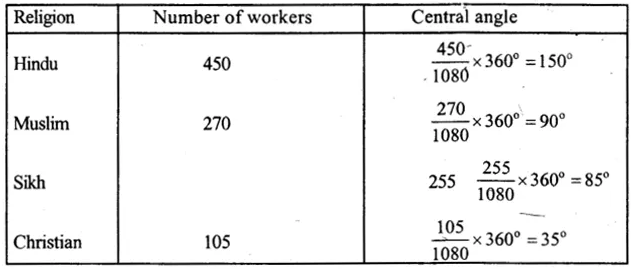Now (i) Draw a circle with a suitable radius
(iii) Starting from this radius, draw sectors whose central angle are 150°, 90°, 85°, 35° respectively.
(iv) Now shade the sectors with different colours or designs as shown in the figure.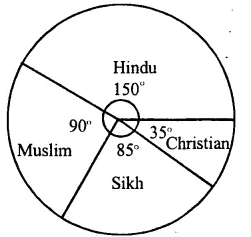Question 6.
Solution:
Total marks obtained by Sudhir
= 105 + 75 + 150 + 120 + 90 = 540(i) Draw a circle with a suitable radius
(iii) Starting from this radius, draw sectors whose central angles are 70°, 50°, 100°, 80° and 60° respectively
(iv) Now shade these sectors with different colours or designs as shown in the figure.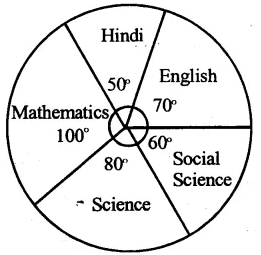Question 7.
Solution:
Total number of fruits = 26 + 30 + 21 + 5 + 8 = 90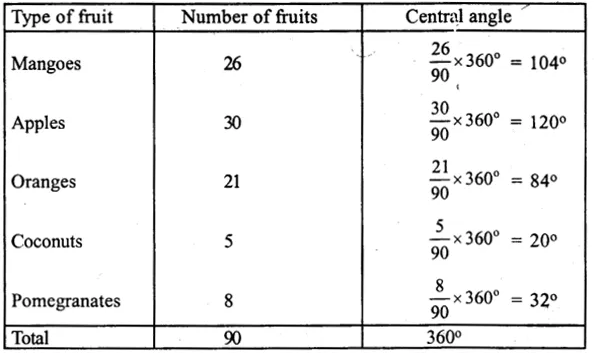(i) Draw a circle with a suitable radius.
(iii) Starting from this radius, draw sectors of central angles 104°, 120°, 84°, 20° and 32° respectively.
(iv) Shade these sectors with different colours or designs as shown in the figure.Question 8.
Solution:
Total number of million of tonnes of food grains = 57 + 76 + 38 + 19 = 190 million of tonnes(i) Draw a circle with suitable radius.
(iii) Starting with this radius, draw sectors of central angles 108°, 144°, 72° and 366 respectively.
(iv) Shade these sectors with different colours or designs as shown in the figure.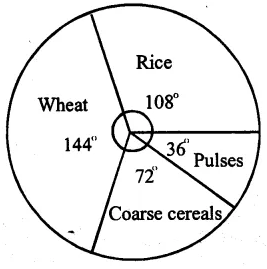Question 9.
Solution:
Total percentage = 25 + 45 + 20 + 10 = 100%(i) Draw a circle with a suitable radius.
(iii) Starting from this radius, draw sectors of central angles 90°, 162°, 72° and 36° respectively.
(iv) Shade these sectors with different colours or designs as shown in the figure.Question 10.
Solution:
Total percentage = 20 + 40 + 25 + 15 = 100%(i) Draw a circle with a suitable radius.
(iii) Starting from this radius, draw sectors of central angles 72°, 144°, 90° and 54° respectively.
(iv) Now shade these sectors with different colours or designs as shown in the figure.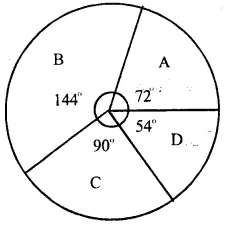Hope given RS Aggarwal Solutions Class 8 Chapter 23 Pie Charts Ex 23A are helpful to complete your math homework.

If you have any doubts, please comment below. Learn Insta try to provide online math tutoring for you.

## RS Aggarwal Class 8 Solutions Chapter 22 Constructing and Interpreting Bar Graphs Ex 22

These Solutions are part of RS Aggarwal Solutions Class 8. Here we have given RS Aggarwal Class 8 Solutions Chapter 22 Constructing and Interpreting Bar Graphs Ex 22.

Question 1.
Solution:
We can draw the bar graph by the following steps :
(i) On the graph draw one horizontal line OX and other vertical line OY representing x-axis and y-axis respectively.
(ii) Along x-axis, write the name of subjects taken at uniform gaps.
(iii) Choose the scale = 1 small division = 1 mark
(vi) Then the heights of various bars will be drawn as shown on the graph.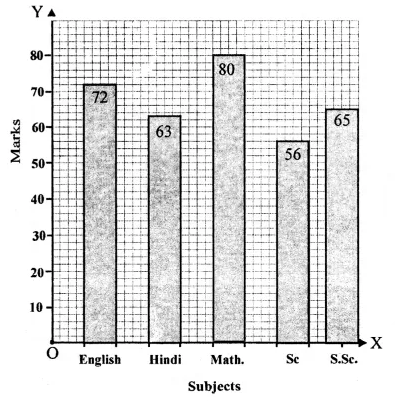Question 2.
Solution:
We can draw the bar graph by the following steps :
(i) On the graph paper, draw a horizontal line OX and another vertical line OY representing x-axis and y-axis respectively.
(ii) Along the x-axis write the years taken on uniform gaps.
(iii) Choose scale : 1 small division = 20 students
(iv) Then the heights of various bars will be drawn as shown on the graph.Question 3.
Solution:
We can draw the bar graph by the following steps :
(i) On the graph paper, draw a horizontal line OX and another vertical line OY representing x-axis and y-axis respectively.
(ii) Along the x-axis, write the names of sports taken on uniform gaps.
(iii) Choose the scale : 1 small division = 1 student
(iv) Then the heights of various bars will be drawn as shown on the graphQuestion 4.
Solution:
We can draw the bar graph by the following steps :
(i) On the graph, draw a horizontal line OX and another vertical line OY representing x-axis and y-axis respectively.
(ii) Along x-axis, write cities with a uniform gaps.
(iii) Choose the scale : 1 small division = 200 km
(iv) Then we shall draw the heights of various bars as shown on the graph.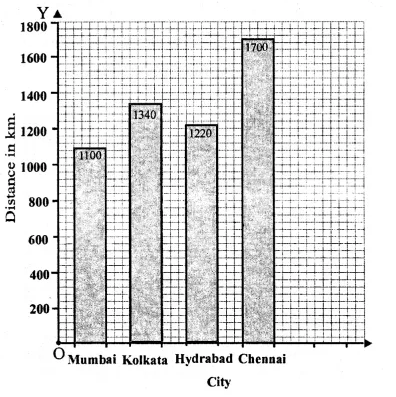Question 5.
Solution:
We can draw the bar graph by the following steps :
(i) On the graph, draw a horizontal line OX and another vertical line OY representing x-axis and y-axis respectively.
(ii) Along x-axis, write countries
(iii) Choose the scale : 1 small division = 10 year
(iv) Then we shall draw the heights of various bars as shown on the graph.Question 6.
Solution:
We can draw a bar graph by the following steps :
(i) On the graph, draw a horizontal line OX and another vertical line OY representing x-axis and y-axis respectively.
(ii) Along x-axis, write modes of transport with uniform gaps.
(iii) Choose the scale : 1 small division = 100 Students
(iv) Then we shall draw the heights of bars as shown on the graph.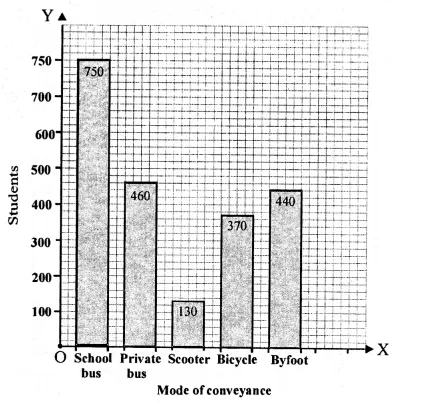Question 7.
Solution:
(i) Draw a horizontal line OX and a vertical line OY which represent x-axis and y-axis respectively on the graph.
(ii) Along OX, write years and along OY, number of motorcycles.
(iii) Choose 1 division = 300
(iv) Now draw bars of different heights according to give data as shown on the graph.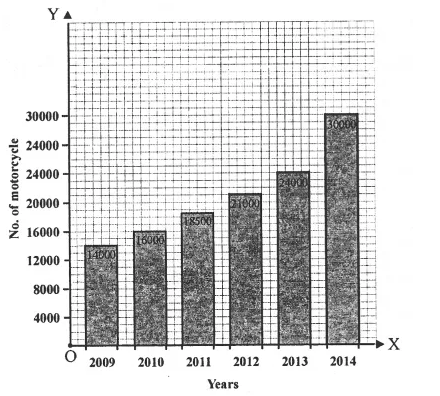Question 8.
Solution:
We can draw the bar graph by the following steps :
(i) On the graph, draw horizontal line OX and another vertical line OY representing x-axis and y-axis respectively.
(ii) Along x-axis, write the names of States given at uniform gaps.
(iii) Choose scale : 1 small division = 200 lakhs
(iv) Then we shall draw the heights of various bars as shown on the graph.Question 9.
Solution:
We can draw the bar graph by the following steps :
(i) On the graph, draw horizontal line OX and another vertical line OY representing x-axis
and y-axis respectively.
(ii) Along x-axis, write the names of animals given at uniform gaps.
(iii) Choose scale : 1 small division = 200 lakhs
(iv) Then we shall draw the heights of various bars as shown on the graph.Question 10.
Solution:
We can draw the bar graph by the following steps :
(i) On the graph paper, draw a horizontal line OX and another vertical line OY representing x-axis and y-axis respectively.
(ii) Along the x-axis write the years taken on uniform gaps.
(iii) Choose scale : 1 small division = 20 export earnings
(iv) Then the heights of various bars will be drawn as shown on the graph.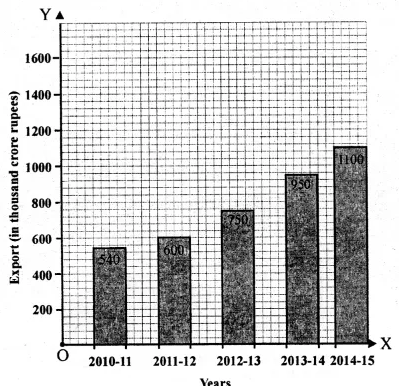Question 11.
Solution:
We can draw the bar graph by the following steps :
(i) On the graph, draw horizontal line OX and another vertical line OY representing x-axis and y-axis respectively.
(ii) Along x-axis, write the names of years given at uniform gaps.
(iii) Choose scale 1 small division = 200 lakhs
(iv) Then we shall draw the heights of various bars as shown on the graph.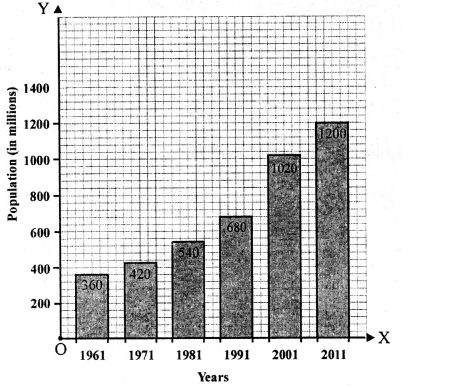Question 12.
Solution:
(i) The bar graph shows the number of members in each of the 100 families of a village.
(ii) 90
(iii) 65
(iv) 5

Question 13.
Solution:
(i) The given bar graph shows the marks obtained by a student in an examination in each of the five subjec ts.
(ii) English.
(iii) From the given graph,
(iv) Mathematics.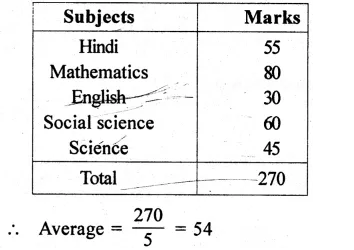Question 14.
Solution:
(i) Mount Everest is the heighest peak and its heights is 8800 m.
(ii) Highest peak is Mount Everest and lowest peak is Annapurna and their heights are 8800 m and 6000 m respectively.
Ratio = 8800 : 6000 => 22 : 15
(iii) Heights of peaks in ascending order is 6000 m, 7500 m, 8000 m, 8200 m and 8800 m.
(iv) Kanchenjunga peak differ by 600 meter from Mount Everest.

Hope given RS Aggarwal Class 8 Solutions Chapter 22 Constructing and Interpreting Bar Graphs Ex 22 are helpful to complete your math homework.

If you have any doubts, please comment below. Learn Insta try to provide online math tutoring for you.

## RS Aggarwal Class 8 Solutions Chapter 21 Data Handling Ex 21B

These Solutions are part of RS Aggarwal Solutions Class 8. Here we have given RS Aggarwal Solutions Class 8 Chapter 21 Data Handling Ex 21B.

Other Exercises

Question 1.
Solution:
Frequency distribution table is given below: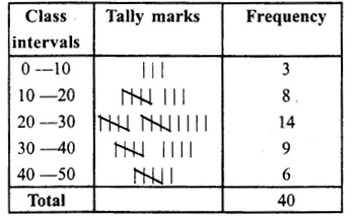Question 2.
Solution:
Arranging the given data in increasing order:
312, 324, 356, 365, 378, 400, 435, 472, 506, 548, 565, 570, 584, 596, 617, 630, 674, 685, 700, 736, 745, 754, 763, 776, 780.
Now frequency distribution table is given below :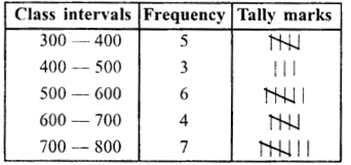Question 3.
Solution:
Frequency Distribution table is given below:Question 4.
Solution:
Frequency distribution table is given belowQuestion 5.
Solution:
Frequency table is given below :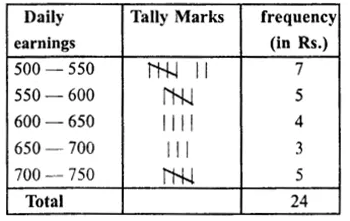Question 6.
Solution: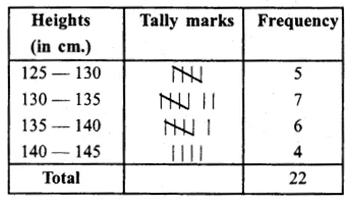Hope given RS Aggarwal Solutions Class 8 Chapter 21 Data Handling Ex 21B are helpful to complete your math homework.

If you have any doubts, please comment below. Learn Insta try to provide online math tutoring for you.

## RS Aggarwal Class 8 Solutions Chapter 21 Data Handling Ex 21A

These Solutions are part of RS Aggarwal Solutions Class 8. Here we have given RS Aggarwal Solutions Class 8 Chapter 21 Data Handling Ex 21A.

Other Exercises

Question 1.
Solution:Question 2.
Solution:Question 3.
Solution: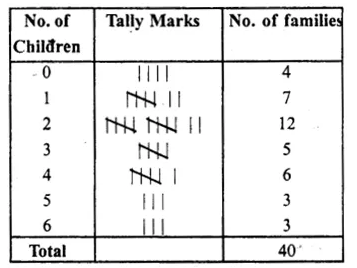Hope given RS Aggarwal Solutions Class 8 Chapter 21 Data Handling Ex 21A are helpful to complete your math homework.

If you have any doubts, please comment below. Learn Insta try to provide online math tutoring for you.

## RS Aggarwal Class 8 Solutions Chapter 20 Volume and Surface Area of Solids Ex 20C

These Solutions are part of RS Aggarwal Solutions Class 8. Here we have given RS Aggarwal Solutions Class 8 Chapter 20 Volume and Surface Area of Solids Ex 20C.

Other Exercises

Tick the correct answer in each of the following:

Question 1.
Solution:
Length (l) = 12 cm
height (h) = 8 cm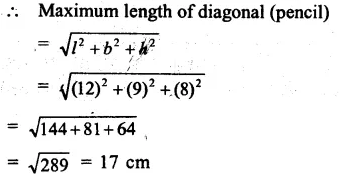Question 2.
Solution:
Total surface area of cube = 150 cm2
Side = $$\sqrt { \frac { 150 }{ 6 } }$$
= √25
= 5 cm
Volume = (side)3
= (5)3
= 125 cm3 (b)

Question 3.
Solution:
Volume of cube = 343 cm2
Side = $$\sqrt [ 3 ]{ 343 } =\sqrt [ 3 ]{ 7\times 7\times 7 }$$
= 7 cm
Total surface area = 6 (side)2
= 6 x (7)2
= 6 x 49 cm2
= 294 cm2 (c)

Question 4.
Solution:
Rate of painting = 10 paise per cm2
Total cost = Rs. 264.60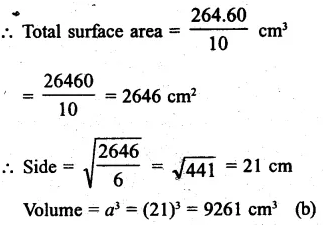Question 5.
Solution:
Length of wall (l) = 8m = 800 cm
Height (h) = 6 m
= 600 cm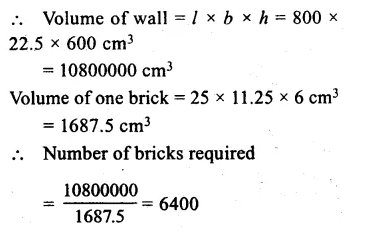Question 6.
Solution:
Edge of cube = 10 cm
Volume = a3 = (10)3 = 1000 cm3
Edge of box = 1 m = 100 cmQuestion 7.
Solution:
Ratio in sides of a cuboid = 1 : 2 : 3
Surface area = 88 cm2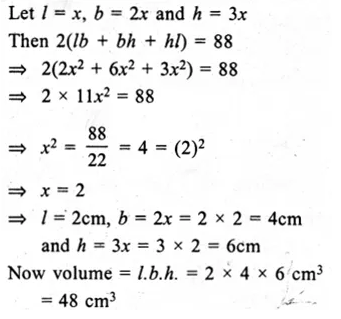Question 8.
Solution:
Ratio in the two volumes = 1 : 27
Let volume of first volume = x3
and volume of second volume = 27x3
Side of first cube = x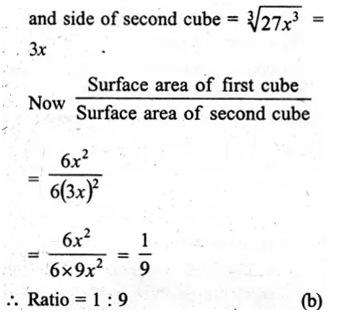Question 9.
Solution:
Surface area of a brick of measure 10 cm x 4 cm x 3 cm
= 2 (l x b + b x h + h x l)
= 2 [10 x 4 + 4 x 3 + 3 x 10] cm2
= 2 [40 + 12 + 30]
= 82 x 2
= 164 cm2 (c)

Question 10.
Solution:
Length of beam (l) = 9 m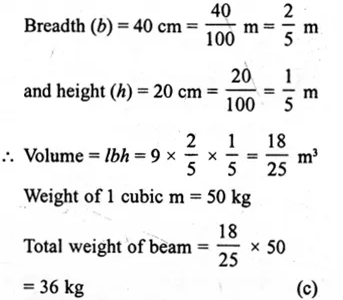Question 11.
Solution:
Water in rectangular reservoir = 42000
Volume = $$\\ \frac { 42000 }{ 1000 }$$ = 42 m3
Length (l) = 6 m
Depth = $$\\ \frac { volume }{ l\times b }$$
= $$\\ \frac { 42 }{ 6\times 3.5 }$$
= 2 m (c)

Question 12.
Solution:
Dimensions of a room are 10 m, 8 m, 3.3 m
Volume of air in it = lbh
= 10 x 8 x 3.3 = 264 m3
Air required for one man = 3 m3
No. of men = $$\\ \frac { 264 }{ 3 }$$
= 88 (b)

Question 13.
Solution:
Length of water tank (l) = 3 m
Width (b) = 2 m
and height (h) = 5 m
Volume = lbh = 3 x 2 x 5 = 30 m3
Water in it = 30 x 1000
= 30000 (a)

Question 14.
Solution:
Size of box = 25 cm, 15 cm, 8 cm
Surface area = (lb + bh + hl)
= 2 ( 25 x 15 + 15 x 8 + 8 x 25) cm2
= 2 (375 + 120 + 200) cm2
= 2(695)
= 1390 cm(b)

Question 15.
Solution:
Diagonal of cube = 4√3
Side = $$\frac { 4\sqrt { 3 } }{ \sqrt { 3 } }$$
= 4 cm
Volume = a3 = (4)3
= 64 cm3 (d)

Question 16.
Solution:
Diagonal of cube = 9√3 cm
Side = $$\frac { 9\sqrt { 3 } }{ \sqrt { 3 } }$$
= 9 cm
Surface area = 6a2
= 6 (9)2 = 6 x 81 cm2
= 486 cm2 (b)

Question 17.
Solution:
Let side of cube in first case = a
Then volume = a3
If side of cube is doubled, then side = 2a
Volume (2a)3 = 8a3
Becomes 8 times (d)

Question 18.
Solution:
Let side of cube in first case = a
Then surface area = 6a2
and side of second cube = 2a
Surface area = 6 (2a)2 = 6 x 4a2 = 24a2
Ratio = $$\frac { { 24a }^{ 2 } }{ { 6a }^{ 2 } }$$ = 4
Becomes 4 times (b)

Question 19.
Solution:
Sides (edges) of 3 cubes are 6 cm, 8 cm, and 10 cm respectively
Volume of first cube = (6)3 = 216 cm3
Volume of second cube = (8)3 = 512 cm3
and volume of third cube
= (10)3 = 1000 cm3
Sum of volumes of 3 cubes = 216 + 512 + 1000
= 1728 cm3
Volume of new single cube = 1728 cm3
Edge = $$\sqrt [ 3 ]{ 1728 }$$
$$\sqrt [ 3 ]{ { \left( 12 \right) }^{ 3 } }$$
= 12 cm (a)

Question 20.
Solution:
Each edge of 5 cubes = 5 cm
Placing than adjacent to each other
Length of new cuboid (l)
= 5 x 5 = 25 cm
and height (h) = 5 cm
Volume of new cuboid = lbh
= 25 x 5 x 5 cm3
= 625 cm3 (d)

Question 21.
Solution:
Diameter of circular well = 2n
Radius = $$\\ \frac { 2 }{ 2 }$$ = 1 m
Depth(h) = 14 m
Volume of earth dug out = πr2h
= $$\\ \frac { 22 }{ 7 }$$ x 1 x 1 x 14
= 44 m (d)

Question 22.
Solution:
Capacity of cylindrical tank = 1848 m3
Diameter = 14 m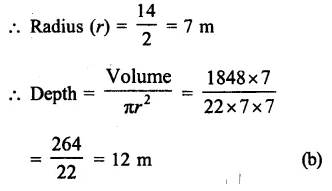Question 23.
Solution:
Radius of a cylinder (r) = 20 cm
and height (h) = 60 cmQuestion 24.
Solution:
Radius of each coin (r) = 0.75 cm
and thickness (h) = 0.2 cm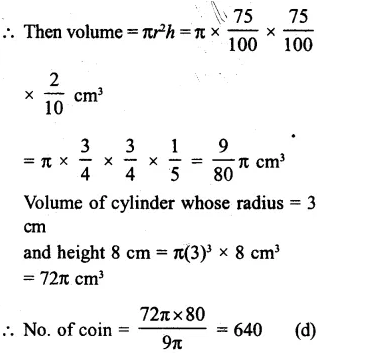Question 25.
Solution:
Volume of silver = 66 cm3
Diameter of wire = 1 mm = $$\\ \frac { 1 }{ 10 }$$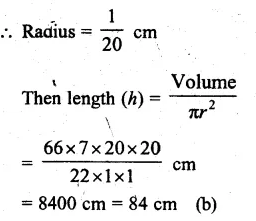Question 26.
Solution:
Diameter of cylinder = 10 cm
Radius (r) = $$\\ \frac { 10 }{ 2 }$$ = 5 cmQuestion 27.
Solution:
Diameter of cylinder = 7 cm
Radius (r) = $$\\ \frac { 7 }{ 2 }$$ cm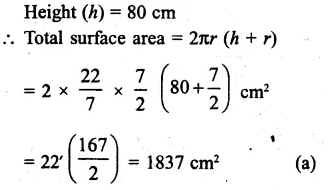Question 28.
Solution:
Curved surface area of a cylinder = 264 cm3
Height (h) = 14 cm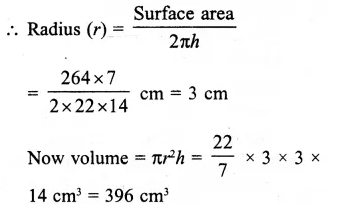Question 29.
Solution:
Diameter of cylinder = 14 cm
Curved surface area = 220 cm2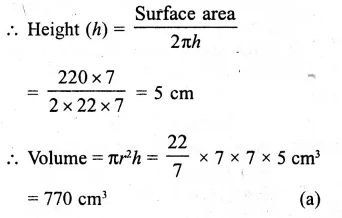Question 30.
Solution:
Ratio in radii of two cylinder = 2 : 3
and ratio in their height = 5 : 3
Let radii of two cylinder = 2x and 3x
and corresponding heights = 5y, 3y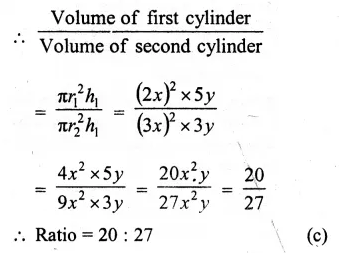Hope given RS Aggarwal Solutions Class 8 Chapter 20 Volume and Surface Area of Solids Ex 20C are helpful to complete your math homework.

If you have any doubts, please comment below. Learn Insta try to provide online math tutoring for you.